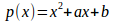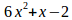Question Pool Polynomials

# Polynomials - SAMAGRA Question Pool & Answers | Class 9 English Medium

Kerala Syllabus SAMAGRA SCERT SAMAGRA Question Pool for Class 9 English Medium Maths PolynomialsQn 1.

The breadth of a rectangle is x, and its length is 2x+5

a)Find its perimeter?

b) Find its area?

c) If the breadth is 5 cm what is its area?

Get Free Study Materials + 1 Week Free Trial of BrainsPrep Class 9 English Medium Tuition

Qn 2.

InIf, p(1)=4and p(2)=9

a) Write p(x)

b) Find p(3)

Get Free Study Materials + 1 Week Free Trial of BrainsPrep Class 9 English Medium Tuition

Qn 3.

The length of a rectangle is 3x+2 and its breadth is 2x-1

a) Find its Perimeter?

b) Calculate its area?

Get Free Study Materials + 1 Week Free Trial of BrainsPrep Class 9 English Medium Tuition

Qn 4.

a)Perimeter = 2(3x+2+2x-1)=10x+2

b) area = (3x+2)(2x-1)=